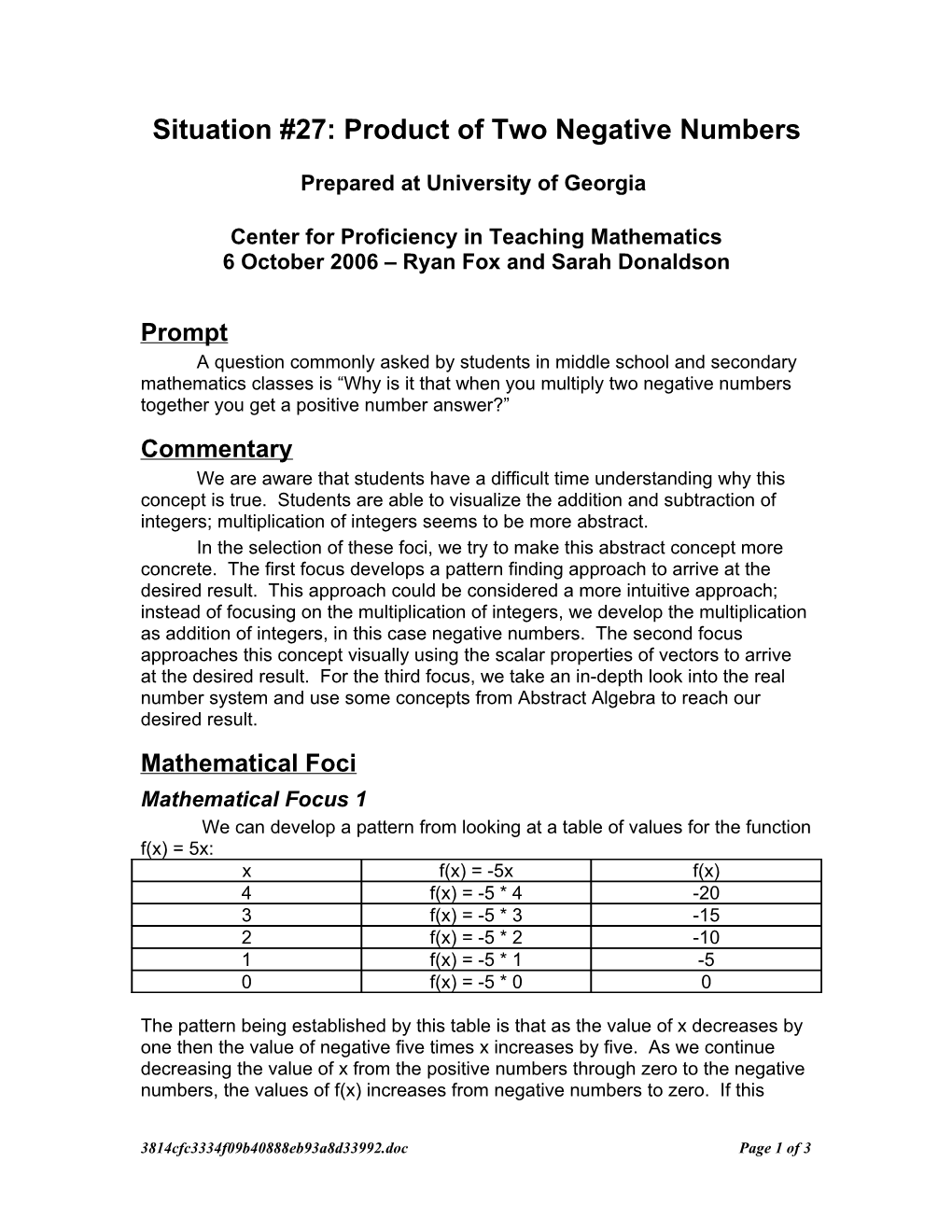# Situation #27: Product of Two Negative NumbersSituation #27: Product of Two Negative Numbers

Prepared at University of Georgia

Center for Proficiency in Teaching Mathematics

6 October 2006 – Ryan Fox and Sarah Donaldson

## Prompt

A question commonly asked by students in middle school and secondary mathematics classes is “Why is it that when you multiply two negative numbers together you get a positive number answer?”

## Commentary

We are aware that students have a difficult time understanding why this concept is true. Students are able to visualize the addition and subtraction of integers; multiplication of integers seems to be more abstract.

In the selection of these foci, we try to make this abstract concept more concrete. The first focus develops a pattern finding approach to arrive at the desired result. This approach could be considered a more intuitive approach; instead of focusing on the multiplication of integers, we develop the multiplication as addition of integers, in this case negative numbers. The second focus approaches this concept visually using the scalar properties of vectors to arrive at the desired result. For the third focus, we take an in-depth look into the real number system and use some concepts from Abstract Algebra to reach our desired result.

## Mathematical Foci

### Mathematical Focus 1

We can develop a pattern from looking at a table of values for the function f(x) = 5x:

x / f(x) = -5x / f(x)
4 / f(x) = -5 * 4 / -20
3 / f(x) = -5 * 3 / -15
2 / f(x) = -5 * 2 / -10
1 / f(x) = -5 * 1 / -5
0 / f(x) = -5 * 0 / 0

The pattern being established by this table is that as the value of x decreases by one then the value of negative five times x increases by five. As we continue decreasing the value of x from the positive numbers through zero to the negative numbers, the values of f(x) increases from negative numbers to zero. If this pattern holds for functional values, then f(x) will be a positive value when x is negative.

x / f(x) = -5x / f(x)
1 / f(x) = -5 * 1 / -5
0 / f(x) = -5 * 0 / 0
-1 / f(x) = -5 * -1 / 5
2 / f(x) = -5 * -2 / 10

We can see that, for example –5 * -2 = 10, the product of two negative numbers is a positive number.

### Mathematical Focus 2

For this focus, we will look at vectors on a number line. Each time a vector is multiplied together with a scalar –1, the vector is reflected about the origin.

A second reflection, as represented by multiplying this new vector by –1, would yield another reflection about the origin again.

The product of the two reflections is the original vector. Therefore we can say that –1 x –1 = 1. If we continue this logic to any two negative scalars, we can show that the product of two scalars, or the product of two negative numbers, is positive.

We can use Geometer’s Sketchpad to show how this idea can be extended to 2 dimensions. For this exploration (which could be accessed by clicking the link to a Sketchpad document), we will declare that a positive direction is represented by a counter-clockwise movement. If we construct a unit square along the postive x and y axes (as seen in the top right yellow square), the point moving represents counter-clockwise movement. When we reflect the square about the y-axis (as seen in the top left blue square), we change the signs of the x-coordinate. As a result of the reflection, the moving point is now going in a clockwise direction, symbolizing a negative orientation. If we reflect the new square about the x axis, we will end up changing the signs of the y-values. However, upon reflection about the x-axis, we see that the point moves in a counter-clockwise direction, thereby signifying a positive orientation. The reflection about the two different axes, each of which involving a change in signs or multiplying by a negative number, yielded a positive orientation.

### Mathematical Focus 3

We can approach this problem using a sophisticated approach to the real number system. The set of real numbers forms a field under the operations of addition and multiplication as they are typically defined. Since we have a field, we are guaranteed additive identities and additive inverses. In addition, the distributive property of multiplication over addition holds. We will use these properties to illustrate that the product of two negative numbers is actually positive. When we refer to negative numbers, we are talking about the additive inverses of natural numbers. Zero acts as the additive identity to this field. We will pick any element from the set of real numbers and call it a.

SitnProdTwoNeg061006.docPage 1 of 3